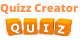Create Quiz

# Laws Of Motion Quiz## Quiz yourself on how well you understand Newton's Laws of Motion, Newton's laws of motion are three physical laws that, together, laid the foundation for classical mechanics. They describe the relationship between a body and the forces acting upon it, and its motion in response to those forces

You can mute/unmute sounds from here

## How long will an object remain at rest, according to Newton's First Law of Motion?

It can't rest.
Until noon.
Until an unbalanced force occurs.
As long as there are unbalanced forces acting on it.

## What is inertia?

The tendency of an object to continue doing what it is currently doing.
The opposing force of gravity.
Another name for unbalanced forces.
The mass of an object.

## Which of Newton's Laws describes the amount of force applied to accelerate an object's mass?

Newton's First Law
Newton's Second Law
Newton's Third Law
Newton's Fourth Law

## For every ________ there is an equal and opposite _____________.

Reaction, action
Action, action
Reaction, reaction
Action, reaction

Newtons
Kilometers
Seconds
Grams

Frictional
Non-contact
Contact
Momentum

## A person is measured on the moon, and on Earth. Which statement would be true?

The person's mass on Earth would be double on the moon.
The person's mass on the the moon, would be twice that on Earth.
The person would have mass on Earth but not on the moon.
The mass would be the same on the moon as it is on Earth.

## How could you tell if a moving object is receiving an unbalanced force?

It might speed up.
It might slow down.
It might change direction.
It might do any of the three things mentioned above.

## The amount of force that gravity has on an object is affected by what factors?

The object's mass.
The object's distance from the gravitational force.
The object's height.
Both A and B.

## Why is it harder to push a van filled with bowling balls than it is to push van filled with basketballs?

The mass of the car is greater than the mass of the basketball.
The mass of the bowling balls is greater than the mass of the basketballs.
The van with the basketballs is bigger than the van with the bowling balls.
It should be equally as hard to push the two vans.

Currently, we have no comments. Be first to comment on this quiz.

SIMILAR QUIZZESPhysics quiz 10
natural science that studies matterPhysics quiz 25
Physics is the branch of science that deals with the structure of matter and how the fundamental constituents of the universe interac

## Laws Of Motion Quiz : Test Trivia

Ultimate impossible quiz game

Embed This Quiz
Copy the code below to embed this quiz